Recipe: Measuring growth between time periods

Follow

You can create reports to demonstrate the growth or decline of your results over time. Using calculated metrics, you can compare results from the current week, month, quarter, or year to the previous time period. This type of calculation is referred to as a X to date comparison .

This recipe will show you how to create a KPI chart displaying the changes in results from the current to previous time period.

There are two methods for creating these calculations:

Creating X to date comparisons for beginning users

This method provides an easy way to create your comparison, but requires more time and calculated elements. If you are looking for a faster, but more advanced method, see Creating X to date comparisons for advanced users .

This example calculates monthly change, but you can use this recipe for weekly, quarterly, or yearly changes.

Time : 15 minutes

Skill : Beginner

Ingredients :
• Two date range calculated metrics
• One standard calculated metric

Creating your current time period to today calculation

The first date range calculated metric will total your results from the current week, month, quarter, or year to date. This recipe measures the current month to date.

To create current time period to present date calculation

1. In the Query Builder, click the Calculation engine icon (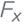).
2. Select Date range calculated metric .
3. Name your metric. This example uses Amount this month.
4. Select the metric you want to view the growth of in the Original metric drop-down list.
5. Select the attribute Date from the Defined on drop-down list.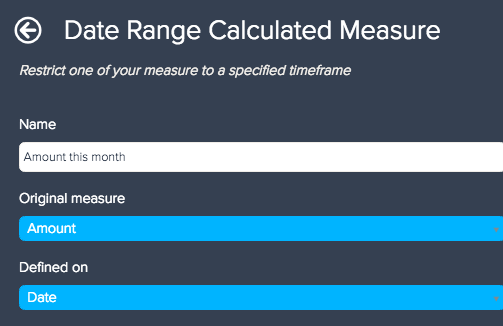7. Under From the beginning of , select the current time period you want to measure from the first option's drop-down list. This example uses This month to calculate results for only the current month.
8. Under To the end of , select Today from the first option's drop-down list.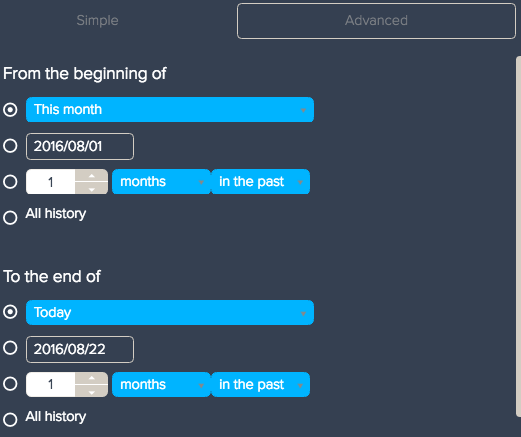9. Click Save .
10. Click the + button on Metrics and add your new date range calculated metric.

Your results should reflect the total from the beginning of your selected time period to the current date. In the example below the current date is 2016-08-22. The results include the beginning of August to the current date.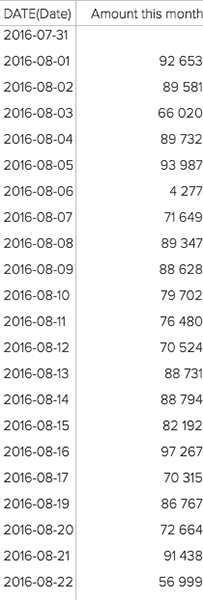Creating your current and previous time period to date calculation

To make sure your comparison stays consistent with your updated time periods, you will need to create a second date range calculated metric to calculate the past month and current month results to the present date. It is important to use a date range calculated metric, so results recalculate when dates change.

To calculate the current and previous time period to date

1. In the Calculation engine menu, select Date range calculated metric .
2. Name your metric. This example uses the name Amount this and previous month.
3. Select the same original metric used in the first date range calculated metric above.
4. Select the Date attribute from the Defined on drop-down list.
6. Select the same settings you used in the previous date range calculated metric.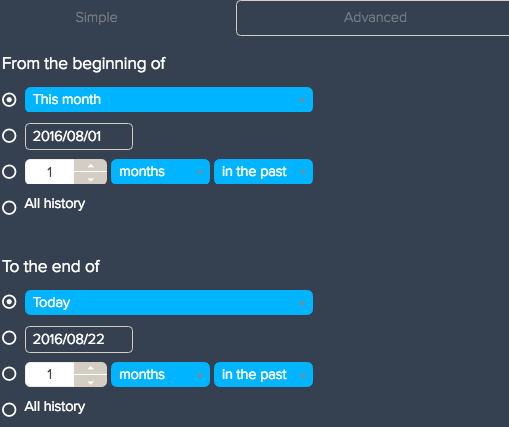7. Under Repeat for , click Add repeat pattern .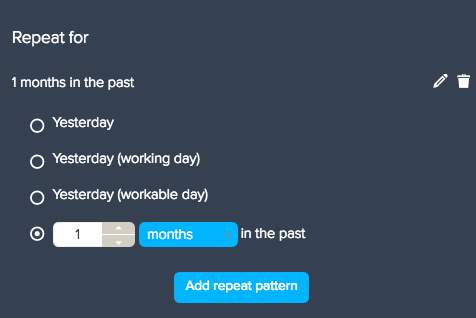8. Enter how far in the past you want to compare to the current time period. Since this example is analyzing results by monthly basis, the repeat pattern is for one month in the past.
9. Click Save .

Your results will now calculate the previous and current month results.

To measure the growth between current and previous results, you need to create a third calculated metric. This calculated metric will provide you with the difference between the results.

1. In the Calculation engine menu, select Standard calculated metric .
2. Name your calculated metric. This example uses the name Last month to date.
3. Subtract your first calculated metric from your second. The formula for the example above would look like below.
`SUM(Amount this and previous week)-SUM(Amount this month)`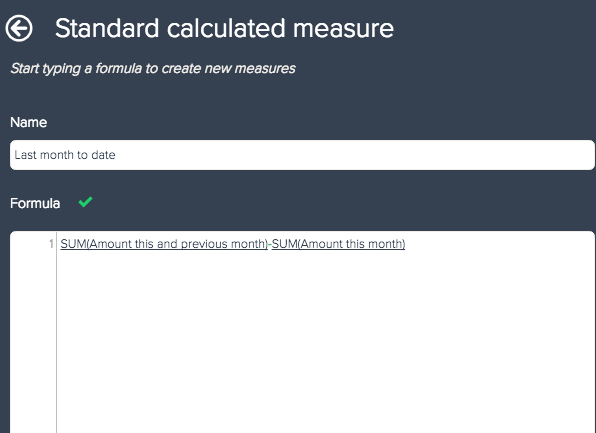4. Click Save .
5. Add your standard calculated metric to your query. Ensure that your standard calculated metric is underneath your current time period to date metric, or the KPI will not compare results properly.

Your KPI should resemble the image below. For information on customizing a KPI chart, see Comparing metrics with KPI .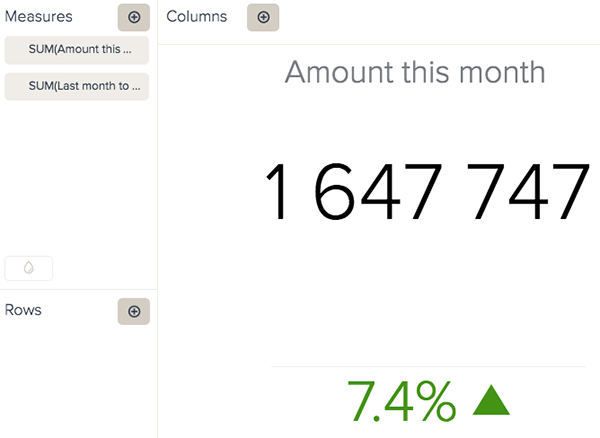Creating X to date comparisons for advanced users

This section contains a more advanced method of creating an X to Date comparison. The advanced metric requires fewer calculated metrics, but a higher experience level.

Note: If you want to create weekly result comparisons using the advanced method, you need to use a web-based service data source .

Time : 10 minutes

Ingredients :
• One date range calculated metric
• One standard calculated metric

Creating your date range calculated metric

Your first calculated metric will calculate results from the beginning of a selected time period to the current date. This example uses a month to date comparison, but you can compare any time period to the current date.
1. In the Query Builder, click the Calculation engine icon ().
2. Select Date range calculated metric .
3. Select your metric for analyzing results from the Original metric drop-down list.
4. Select the Date attribute from the Defined on drop-down list.
5. In the first option for From beginning of , select the time period you want to use to measure to your current date. This example uses This month to calculate results from the current month to today.
6. Under To the end of , select Today from the drop-down list.
7. Click Save .
8. Add your date range calculated metric to your report by clicking the + button on Metrics.

Your query will display the results of your selected time period to the current date.

Unlike the beginner method above, you do not need to create a second date range calculated metric to measure your growth. Depending on the starting time period you selected, your standard calculated metric formula will be different.
1. In the Calculation engine menu, select Standard calculated metric .
2. Name your calculated metric. This example uses Month to date.
3. Enter one of the following formulas. You will use the formula for the time period you are comparing.
• Enter the following formula if you are comparing weekly:
`IF (DATE_GREATER_OR_EQUAL([DATE(Date)], START_OF_WEEK_MONDAY(DATE_ADD(TODAY(),"day",-7))) AND DATE_LESS_OR_EQUAL([DATE(Date)],DATE_ADD(TODAY(),"day",-7))) THEN SUM(Amount) ELSE 0 ENDIF`
• Enter the following formula if you are comparing monthly:
`IF (DATE_GREATER_OR_EQUAL([DATE(Date)], START_OF_MONTH(DATE_ADD(TODAY(),"month",-1)))AND DATE_LESS_OR_EQUAL([DATE(Date)],DATE_ADD(TODAY(),"month",-1)))THEN SUM(Amount) ELSE 0 ENDIF`
• Enter the following formula if you are comparing yearly:
`IF (DATE_GREATER_OR_EQUAL([DATE(Date)],  DATE(YEAR(TODAY())-1,1,1,0,0,0)) AND DATE_LESS_OR_EQUAL([DATE(Date)],DATE_ADD(TODAY(),"year",-1)))THEN SUM(Amount) ELSE 0 ENDIF`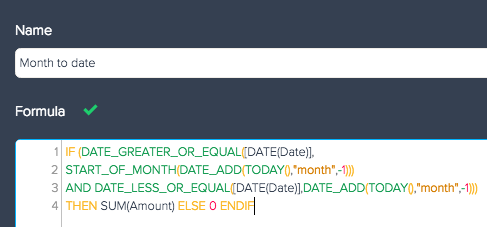4. Click Save .

Your report should resemble the image below. For information on customizing a KPI chart, see Comparing metrics with KPI .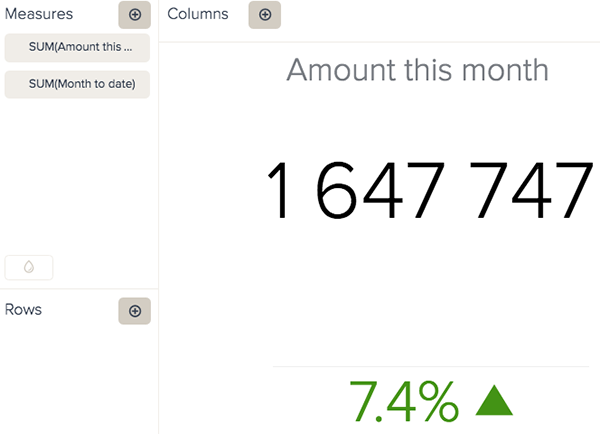2 out of 2 found this helpful
Have more questions? Submit a request

• Dude this is awesome thank you. just wanted to point out at type with the weekly formula. just missing a couple of parenthesis.

IF (DATE_GREATER_OR_EQUAL([DATE(Date)],